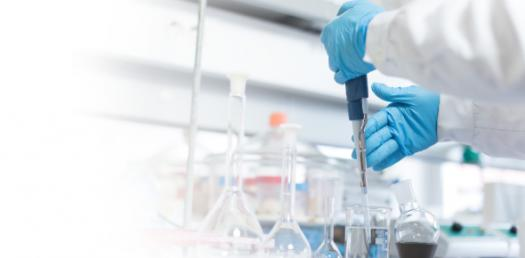Quiz: Can You Pass Chemical Reaction Trivia Test?

10 Questions | Total Attempts: 56SettingsCan You Pass the Chemical Reaction Trivia Test? Chemical reactions are a result of mixing specific elements, and the solution can be different based on what the mixture is made of. Take up the quiz below and get to see just how knowledgeable you are about chemical elements and the reactions you would expect when you mix them. All the best!

• 1.
61.    What is the molar mass of acetylsalicylic (aspirin) with chemical formula C9H8O4
• A.

29 g/mol

• B.

108 g/mol

• C.

180 g/mol

• D.

196 g/mol

• 2.
In a single replacement reaction, why will one metal replace another metal in an ionic compound?
• A.

The metal will replace another metal if it has a smaller mass and thus is able to hit the ionic compound with a higher velocity and thus more energy.

• B.

The metal will replace another metal if it has a larger mass and thus is able to hit the ionic compound with more energy.

• C.

The metal will replace another metal if it can gain electrons more readily than the other metal.

• D.

The metal will replace another metal if it can lose electrons more readily than the other metal.

• 3.
The simplest whole-number ratio of atoms in a compound is called the ________.
• 4.
BUGOY decided to mix different chemicals at random. Which of the following mixture of solutions should she combine to form a precipitate? (Numbers in the choices should be
• A.

A solution of Na2S and a solution Cu(NO3)2

• B.

A solution of Na3PO4 and a solution of KCl

• C.

A solution of KNO3 and a solution of FeCl3

• D.

None of these produce a precipitate

• 5.
To convert between moles and atoms of a substance, _____ must be used.
• 6.
The empirical formula of butane is C2H5. If the molar mass of butane is 58 g/mol, then its molecular formula is _________.
• 7.
For the reaction Na(s) + H­2O àNaOH(aq)  + H2 , which of the following sets of coefficients will balance it?
• A.

1, 1, 2, 2

• B.

2, 2, 2, 1

• C.

1, 1, 1, 2

• D.

2, 1, 1, 2

• 8.
Which of the following statements is true about chemical reactions?
• A.

New atoms are created during chemical reactions.

• B.

The mass of the product is less than the mass of the reactants.

• C.

Atoms rearranged to form new substances.

• D.

All of the above

• 9.
When two ionic compounds are dissolved in water, a double replacement reaction can ________.
• A.

Occur if the products are both aqueous solutions of ionic compounds.

• B.

Occur if two of the ions form an insoluble ionic compound, which precipitates out of solution.

• C.

Occur only if the ions form covalent bonds with each other.

• D.

Never occur if the ions react to form a gas, which will bubble out of the solution.

• 10.
Which of the following substances is a possible product for the decomposition of Nickel (II) chlorate?
• A.

Nickel oxide

• B.

Chlorine gas

• C.

Oxygen gas

• D.

Chlorine dioxide

Related TopicsBack to top